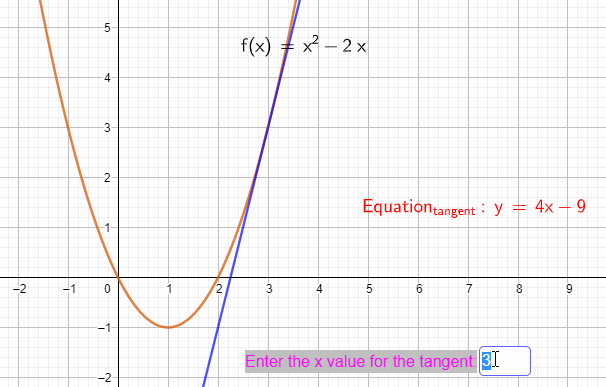### Basic Calculus - differentiation for beginners IGCSE, AS, A-LevelThe process of finding the gradient or slope of a function is the differentiation.

We used to find the gradient of a straight line, just by dividing the change in 'y' by change in 'x', in a certain range of values. When it comes to a curve, however, it is not easy to find the gradient or slope as the very thing we want to measure, keeps changing from point to point.

In the above applet, a tangent is drawn to find the gradient at a given point on the curve. With differentiation, it is just two steps away.

E.g.

Let y = x2

Gradient of the curve at any point = dy/dx = 2x

Gradient at x = 2, dy/dx = 2x2 = 4
Gradient at x = 0, dy/dx = 2x0 = 0
Gradient at x = -3, dy/dx = 2x-3 = -6

For a more detailed tutorial with lots of worked examples on the following, please use this link on the website connected to this blog.

• Finding differential coefficient from the first principles
• Finding the equations of a tangent and a normal to a curve
• Increasing and decreasing functions
• Turning / stationary points
• Local maxima, minima and point of inflection
• Modelling real life situations with differentiation
• Plenty of worked examples and applets for interactive practice